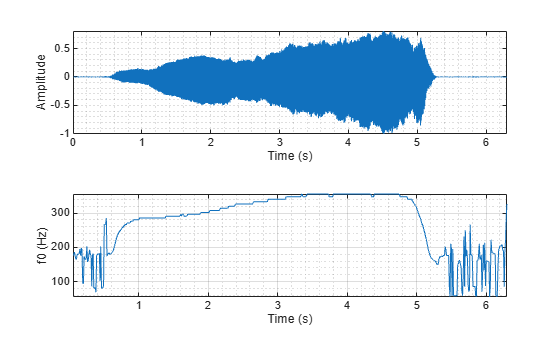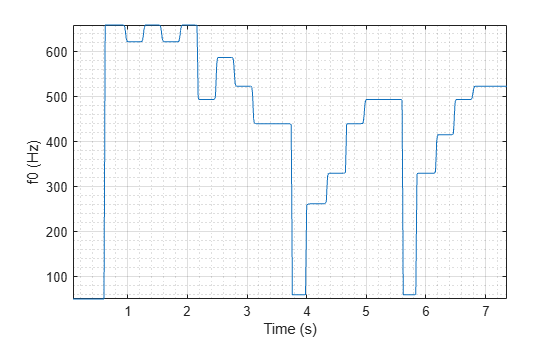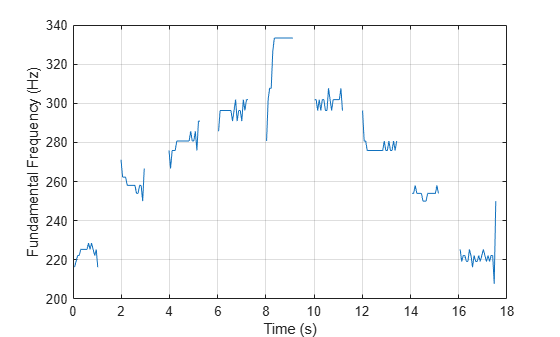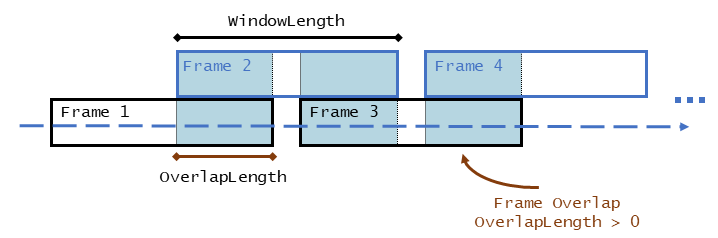# pitch

Estimate fundamental frequency of audio signal

## Syntax

``f0 = pitch(audioIn,fs)``
``f0 = pitch(audioIn,fs,Name,Value)``
``[f0,loc] = pitch(___)``

## Description

example

````f0 = pitch(audioIn,fs)` returns estimates of the fundamental frequency over time for the audio input, `audioIn`, with sample rate `fs`. Columns of the input are treated as individual channels.```

example

````f0 = pitch(audioIn,fs,Name,Value)` specifies options using one or more `Name,Value` pair arguments.```

example

````[f0,loc] = pitch(___)` returns the locations, `loc`, associated with fundamental frequency estimates.```

## Examples

collapse all

Read in an audio file and then call the `pitch` function with default parameters.

```[audioIn,fs] = audioread('Counting-16-44p1-mono-15secs.wav'); [f0,idx] = pitch(audioIn,fs);```

Plot the audio signal and pitch contour.

```subplot(2,1,1) plot(audioIn) ylabel('Amplitude') subplot(2,1,2) plot(idx,f0) ylabel('Pitch (Hz)') xlabel('Sample Number')```The `pitch` function estimates the fundamental frequency of the input signal at locations determined by the `WindowLength` and `OverlapLength` name-value pairs.

Load an audio file of the introduction to Für Elise and the sample rate of the audio. Call the `pitch` function using the pitch estimate filter (`PEF`), a search range from 50 Hz to 800 Hz, a window length of 80 ms, and an overlap of 50 ms. Plot the results and listen to the song to verify the fundamental frequency estimates returned by the `pitch` function.

```load FurElise.mat song fs [f0,loc] = pitch(song,fs, ... 'Method','PEF', ... 'Range',[50 800], ... 'WindowLength',round(fs*0.08), ... 'OverlapLength',round(fs*0.05)); t = loc/fs; plot(t,f0) ylabel('Pitch (Hz)') xlabel('Time (s)')````sound(song,fs)`

The different methods of estimating pitch provide trade-offs in terms of noise robustness, accuracy, optimal lag, and computation expense. In this example, you compare the performance of different pitch detection algorithms in terms of gross pitch error (GPE) and computation time under different noise conditions.

Prepare Test Signals

Load an audio file and determine the number of samples it has. Also load the true pitch corresponding to the audio file. The true pitch was determined as an average of several third-party algorithms on the clean speech file.

```[audioIn,fs] = audioread('Counting-16-44p1-mono-15secs.wav'); numSamples = size(audioIn,1); load TruePitch.mat truePitch```

Create test signals by adding noise to the audio signal at given SNRs. The `mixSNR` function is a convenience function local to this example, which takes a signal, noise, and requested SNR and returns a noisy signal at the request SNR.

```testSignals = zeros(numSamples,4); turbine = audioread('Turbine-16-44p1-mono-22secs.wav'); testSignals(:,1) = mixSNR(audioIn,turbine,20); testSignals(:,2) = mixSNR(audioIn,turbine,0); whiteNoiseMaker = dsp.ColoredNoise('Color','white','SamplesPerFrame',size(audioIn,1)); testSignals(:,3) = mixSNR(audioIn,whiteNoiseMaker(),20); testSignals(:,4) = mixSNR(audioIn,whiteNoiseMaker(),0);```

Save the noise conditions and algorithm names as cell arrays for labeling and indexing.

```noiseConditions = {'Turbine (20 dB)','Turbine (0 dB)','WhiteNoise (20 dB)','WhiteNoise (0 dB)'}; algorithms = {'NCF','PEF','CEP','LHS','SRH'};```

Run Pitch Detection Algorithms

Preallocate arrays to hold pitch decisions for each algorithm and noise condition pair, and the timing information. In a loop, call the `pitch` function on each combination of algorithm and noise condition. Each algorithm has an optimal window length associated with it. In this example, for simplicity, you use the default window length for all algorithms. Use a 3-element median filter to smooth the pitch decisions.

```f0 = zeros(numel(truePitch),numel(algorithms),numel(noiseConditions)); algorithmTimer = zeros(numel(noiseConditions),numel(algorithms)); for k = 1:numel(noiseConditions) x = testSignals(:,k); for i = 1:numel(algorithms) tic f0temp = pitch(x,fs, ... 'Range',[50 300], ... 'Method',algorithms{i}, ... 'MedianFilterLength',3); algorithmTimer(k,i) = toc; f0(1:max(numel(f0temp),numel(truePitch)),i,k) = f0temp; end end```

Compare Gross Pitch Error

Gross pitch error (GPE) is a popular metric when comparing pitch detection algorithms. GPE is defined as the proportion of pitch decisions for which the relative error is higher than a given threshold, traditionally 20% in speech studies. Calculate the GPE and print it to the Command Window.

```idxToCompare = ~isnan(truePitch); truePitch = truePitch(idxToCompare); f0 = f0(idxToCompare,:,:); p = 0.20; GPE = mean( abs(f0(1:numel(truePitch),:,:) - truePitch) > truePitch.*p).*100; for ik = 1:numel(noiseConditions) fprintf('\nGPE (p = %0.2f), Noise = %s.\n',p,noiseConditions{ik}); for i = 1:size(GPE,2) fprintf('- %s : %0.1f %%\n',algorithms{i},GPE(1,i,ik)) end end```
```GPE (p = 0.20), Noise = Turbine (20 dB). - NCF : 0.9 % - PEF : 0.4 % - CEP : 8.2 % - LHS : 8.2 % - SRH : 6.0 % GPE (p = 0.20), Noise = Turbine (0 dB). - NCF : 5.6 % - PEF : 24.5 % - CEP : 11.6 % - LHS : 9.4 % - SRH : 46.8 % GPE (p = 0.20), Noise = WhiteNoise (20 dB). - NCF : 0.9 % - PEF : 0.0 % - CEP : 12.9 % - LHS : 6.9 % - SRH : 2.6 % GPE (p = 0.20), Noise = WhiteNoise (0 dB). - NCF : 0.4 % - PEF : 0.0 % - CEP : 23.6 % - LHS : 7.3 % - SRH : 1.7 % ```

Calculate the average time it takes to process one second of data for each of the algorithms and print the results.

```aT = sum(algorithmTimer)./((numSamples/fs)*numel(noiseConditions)); for ik = 1:numel(algorithms) fprintf('- %s : %0.3f (s)\n',algorithms{ik},aT(ik)) end```
```- NCF : 0.045 (s) - PEF : 0.184 (s) - CEP : 0.050 (s) - LHS : 0.151 (s) - SRH : 0.171 (s) ```

Read in an entire speech file and determine the fundamental frequency of the audio using the `pitch` function. Then use the `voiceActivityDetector` to remove irrelevant pitch information that does not correspond to the speaker.

Read in the audio file and associated sample rate.

`[audio,fs] = audioread('Counting-16-44p1-mono-15secs.wav');`

Specify pitch detection using a 50 ms window length and 40 ms overlap (10 ms hop). Specify that the `pitch` function searches for the fundamental frequency over the range 50-150 Hz and postprocesses the results with a median filter. Plot the results.

```windowLength = round(0.05*fs); overlapLength = round(0.04*fs); hopLength = windowLength - overlapLength; [f0,loc] = pitch(audio,fs, ... 'WindowLength',windowLength, ... 'OverlapLength',overlapLength, ... 'Range',[50 150], ... 'MedianFilterLength',3); plot(loc/fs,f0) ylabel('Fundamental Frequency (Hz)') xlabel('Time (s)')```Create a `dsp.AsyncBuffer` System object™ to chunk the audio signal into overlapped frames. Also create a `voiceActivityDetector` System object™ to determine if the frames contain speech.

```buffer = dsp.AsyncBuffer(numel(audio)); write(buffer,audio); VAD = voiceActivityDetector;```

While there are enough samples to hop, read from the buffer and determine the probability that the frame contains speech. To mimic the decision spacing in time of the `pitch` function, the first frame read from the buffer has no overlap.

```n = 1; probabilityVector = zeros(numel(loc),1); while buffer.NumUnreadSamples >= hopLength if n==1 x = read(buffer,windowLength); else x = read(buffer,windowLength,overlapLength); end probabilityVector(n) = VAD(x); n = n+1; end```

Use the probability vector determined by the `voiceActivityDetector` to plot a pitch contour for the speech file that corresponds to regions of speech.

```validIdx = probabilityVector>0.99; loc(~validIdx) = nan; f0(~validIdx) = nan; plot(loc/fs,f0) ylabel('Fundamental Frequency (Hz)') xlabel('Time (s)')```## Input Arguments

collapse all

Audio input signal, specified as a vector or matrix. The columns of the matrix are treated as individual audio channels.

Data Types: `single` | `double`

Sample rate of the input signal in Hz, specified as a positive scalar.

The sample rate must be greater than or equal to twice the upper bound of the search range. Specify the search range using the `Range` name-value pair.

Data Types: `single` | `double`

### Name-Value Pair Arguments

Specify optional comma-separated pairs of `Name,Value` arguments. `Name` is the argument name and `Value` is the corresponding value. `Name` must appear inside quotes. You can specify several name and value pair arguments in any order as `Name1,Value1,...,NameN,ValueN`.

Example: `pitch(audioIn,fs,'Range',[50,150],'Method','PEF')`

Search range for pitch estimates, specified as the comma-separated pair consisting of 'Range' and a two-element row vector with increasing positive integer values. The function searches for a best estimate of the fundamental frequency within the upper and lower band edges specified by the vector, according to the algorithm specified by `Method`. The range is inclusive and units are in Hz.

Valid values for the search range depend on the sample rate, `fs`, and on the values of `WindowLength` and `Method`:

MethodMinimum RangeMaximum Range
'`NCF`'```fs/WindowLength < Range(1)``````Range(2) < fs/2```
'`PEF`'`10 < Range(1)````Range(2) < min(4000,fs/2)```
'`CEP`'```fs/(2^nextpow2(2*WindowLength-1)) < Range(1)``````Range(2) < fs/2```
'`LHS`'`1 < Range(1)````Range(2) < fs/5 - 1```
'`SRH`'`1 < Range(1)````Range(2) < fs/5 - 1```

Data Types: `single` | `double`

Number of samples in the analysis window, specified as the comma-separated pair consisting of '`WindowLength`' and an integer in the range [1, min(size(`audioIn`,1), 192000)]. Typical analysis windows are in the range 20–100 ms. The default window length is 52 ms.

Data Types: `single` | `double`

Number of samples of overlap between adjacent analysis windows, specified as the comma-separated pair consisting of '`OverlapLength`' and an integer in the range (`-inf`,`WindowLength`). A negative overlap length indicates non-overlapping analysis windows.

Data Types: `single` | `double`

Method used to estimate pitch, specified as the comma-separated pair consisting of '`Method`' and `'NCF'`, `'PEF'`,`'CEP'`, `'LHS'`, or `'SRH'`. The different methods of calculating pitch provide trade-offs in terms of noise robustness, accuracy, and computation expense. The algorithms used to calculate pitch are based on the following papers:

• `'NCF'` –– Normalized Correlation Function 

• `'PEF'` –– Pitch Estimation Filter . The function does not use the amplitude compression described by the paper.

• `'CEP'` –– Cepstrum Pitch Determination 

• `'LHS'` –– Log-Harmonic Summation 

• `'SRH'` –– Summation of Residual Harmonics 

Data Types: `char` | `string`

Median filter length used to smooth pitch estimates over time, specified as the comma-separated pair consisting of '`MedianFilterLength`' and a positive integer. The default, `1`, corresponds to no median filtering. Median filtering is a postprocessing technique used to remove outliers while estimating pitch. The function uses `movmedian` after estimating the pitch using the specified `Method`.

Data Types: `single` | `double`

## Output Arguments

collapse all

Estimated fundamental frequency, in Hz, returned as a scalar, vector, or matrix. The number of rows returned depends on the values of the `WindowLength` and `OverlapLength` name-value pairs, and on the input signal size. The number of columns (channels) returned depends on the number of columns of the input signal size.

Data Types: `single` | `double`

Locations associated with fundamental frequency estimations, returned as a scalar, vector, or matrix the same size as `f0`.

Fundamental frequency is estimated locally over a region of `WindowLength` samples. The values of `loc` correspond to the most recent sample (largest sample number) used to estimate fundamental frequency.

Data Types: `single` | `double`

## Algorithms

The `pitch` function segments the audio input according to the `WindowLength` and `OverlapLength` arguments. The fundamental frequency is estimated for each frame. The locations output, `loc` contains the most recent samples (largest sample numbers) of the corresponding frame.For a description of the algorithms used to estimate the fundamental frequency, consult the corresponding references:

• `'NCF'` –– Normalized Correlation Function 

• `'PEF'` –– Pitch Estimation Filter . The function does not use the amplitude compression described by the paper.

• `'CEP'` –– Cepstrum Pitch Determination 

• `'LHS'` –– Log-Harmonic Summation 

• `'SRH'` –– Summation of Residual Harmonics 

 Atal, B.S. "Automatic Speaker Recognition Based on Pitch Contours." The Journal of the Acoustical Society of America. Vol. 52, No. 6B, 1972, pp. 1687–1697.

 Gonzalez, Sira, and Mike Brookes. "A Pitch Estimation Filter robust to high levels of noise (PEFAC)." 19th European Signal Processing Conference. Barcelona, 2011, pp. 451–455.

 Noll, Michael A. "Cepstrum Pitch Determination." The Journal of the Acoustical Society of America. Vol. 31, No. 2, 1967, pp. 293–309.

 Hermes, Dik J. "Measurement of Pitch by Subharmonic Summation." The Journal of the Acoustical Society of America. Vol. 83, No. 1, 1988, pp. 257–264.

 Drugman, Thomas, and Abeer Alwan. "Joint Robust Voicing Detection and Pitch Estimation Based on Residual Harmonics." Proceedings of the Annual Conference of the International Speech Communication Association, INTERSPEECH. 2011, pp. 1973–1976.

##### SupportGet trial now